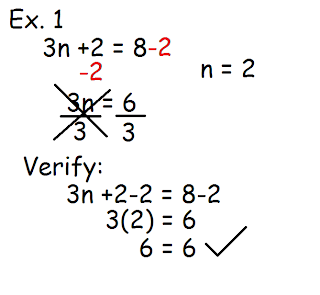## Monday, May 9, 2011

### Jayvee's Algebra Post

How to Solve One Step Algebra Questions:
1) Isolate the variable (the unknown number in an algebra question)
2) Cancel the constant (the integer in an algebra question)
3) Balance by doing the same thing on one side and the other side of the equal sign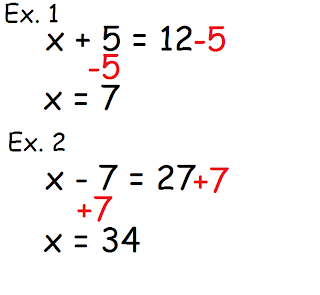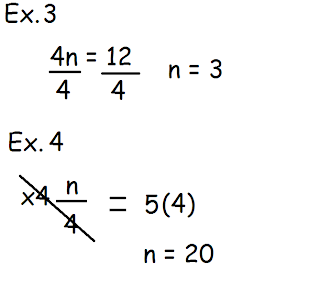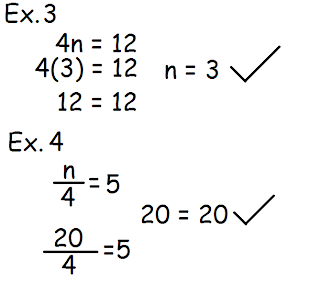Using Alge-Tiles: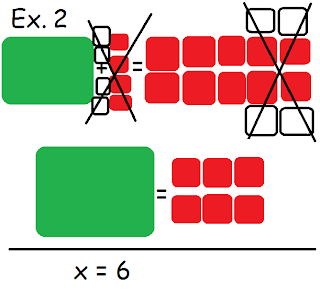How to Solve Two Step Algebra Questions:
1) Isolate the variable (the unknown number in an algebra question)
2) Cancel the constant (the integer in an algebra question)
3) Balance by doing the same thing on one side and the other side of the equal sign
4) Simplify the variable
5) Repeat step #3